×#### Thank you for registering.

One of our academic counsellors will contact you within 1 working day.

Click to Chat

1800-1023-196

+91-120-4616500

CART 0

• 0

MY CART (5)

Use Coupon: CART20 and get 20% off on all online Study Material

ITEM
DETAILS
MRP
DISCOUNT
FINAL PRICE
Total Price: Rs.

There are no items in this cart.
Continue Shopping• Complete JEE Main/Advanced Course and Test Series
• OFFERED PRICE: Rs. 15,900
• View Details

```Chapter 9: Arithmetic Progressions Exercise 9.5

Question: 1

Find the sum of the following arithmetic progressions:

(i) 50, 46, 42, ... to 10 terms

(ii) 1, 3, 5, 7, ... to 12 terms

(iii) 3, 9/2, 6, 15/2, ... to 25 terms

(iv) 41, 36, 31, ... to 12 terms

(v) a + b, a - b, a - 3b, ... to 22 terms

(vi) (x - y)2, (x2 + y2), (x + y)2, to 22 tams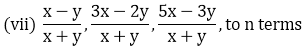(viii) – 26, – 24, – 22, .... to 36 terms

Solution:

In an A.P let first term = a, common difference = d, and there are n terms.

Then, sum of n terms is,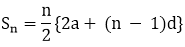(i) Given progression is,

50, 46, 42 to 10 term.

First term (a) = 50

Common difference (d) = 46 - 50 = – 4

nth term = 10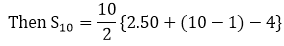= 5{100 - 9.4}

= 5{100 - 36}

= S × 64

∴ S10 = 320

(ii) Given progression is, 1, 3, 5, 7, .....to 12 terms

First term difference (d) = 3 - 1 = 2 nth term = 12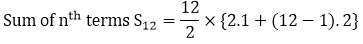= 6 × {2 + 22} = 6.24

∴ S12 = 144.

(iii) Given expression is 3, 9/2, 6, 15/2, ... to 25 terms

First term (a) = 3

Common difference (d) = 9/2 - 3 = 3/2

Sum of nth terms Sn, given n = 25(iv) Given expression is, 41, 36, 31 to 12 terms.

First term (a) = 41

Common difference (d) = 36 - 41 = - 5

Sum of nth terms Sn, given n = 12(v) a + b, a – b, a - 3b to 22 terms

First term (a) = a + b

Common difference (d) = a - b - a - b = -2b

Sum of nth terms Sn = n/2{2a(n - 1). d}

Here n = 22

S22 = 22/2{2.(a + b) + (22 - 1). -2b}

= 11{2(a + b) - 22b)

= 11{2a - 20b}

= 22a - 440b

∴S22 = 22a - 440b

(vi) (x - y)2,(x2 + y2), (x + y)2,... to n terms

First term (a) = (x - y)2

Common difference (d) = x2 + y2 - (x - y)2

= x2 + y2 - (x2 + y2 - 2xy)

= x2 + y2 - x2 + y2 + 2xy

= 2xy

Sum of nth terms Sn = n/2{2a(n - 1). d}

= n/2{2(x - y)2 + (n - 1). 2xy}

= n{(x - y)2 + (n - 1)xy}

∴ Sn = n{(x — y)2 + (n — 1). xy)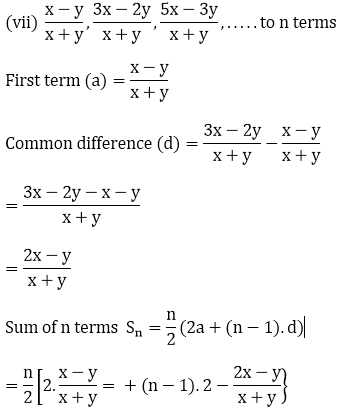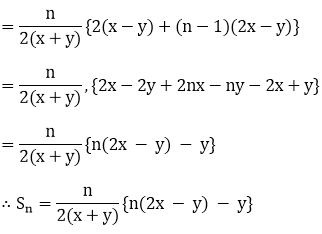(viii) Given expression -26, - 24. -22, to 36 terms

First term (a) = -26

Common difference (d) = -24 - (-26)

= -24 + 26 = 2

Sum of n tams Sn = n/2{2a + (n - 1)d)

Sum of n tams Sn = 36/2{2. -26 + (36 - 1)2}

= 18[-52 + 70]

= 18.18

= 324

∴ Sn = 324

Question: 2

Find the sum to n tam of the A.P. 5, 2, –1, – 4, –7, ...

Solution:

Given AP is 5, 2, -1, -4, -7, .....

a = 5, d = 2 - 5 = -3

Sn = n/2{2a + (n - 1)d}

= n/2{2.5 + (n - 1) - 3}

= n/2{10 - 3(n - 1)}

= n/2{13 - 3n)

∴ Sn = n/2(13 - 3n)

Question: 3

Find the sum of n terms of an A.P. whose the terms is given by an = 5 - 6n.

Solution:

Given nth term an = 5 - 6n

Put n = 1, a1 = 5 - 6.1 = -1

We know, first term (a1) = -1

Last term (an) = 5 - 6n = 1

Then Sn = n/2(-1 + 5 - 6n)

= n/2(4 - 6n) = n/2(2 - 3n)

Question: 4

If the sum of a certain number of terms starting from first term of an A.P. is 25, 22, 19, ... is 116. Find the last term.

Solution:

Given AP is 25, 22, 19, ..........

First term (a) = 25, d = 22 - 25 = -3.

Given, Sn = n/2(2a + (n - 1)d)

116 = n/2(2 × 25 + (n - 1) - 3)

232 = n(50 - 3(n - 1))

232 = n(53 - 3n)

232 = 53n - 3n2

3n2 - 53n + 232 = 0

(3n - 29)(n - 8) = 0

⟹ ag = 25 + (8 - 1) – 3

∴ n = 8, ag = 4

= 25 - 21 = 4

Question: 5

(i) How many terms of the sequence 18, 16, 14, ... should be taken so that their

(ii) How many terms are there in the A P. whose first and fifth terms are -14 and 2 respectively and the sum of the terms is 40?

(iii) How many terms of the A.P. 9, 17, 25, . must be taken so that their sum is 636?

(iv) How many terms of the A P. 63, 60, 57, ... must be taken so that their sum is 693?

Solution:

(i) Given sequence, 18, 16, 14, ...

a = 18, d = 16 - 18 = -2.

Let, sum of n terms in the sequence is zero

Sn = 0

n/2(2a + (n. - 1)d) = 0

n/2(2.18 + (n - 1) - 2) = 0

n(18 - (n - 1)) = 0

n(19 - n) = 0

n = 0 or n = 19

(ii) ∵ n = 0 is not possible.

Therefore, sum of 19 numbers in the sequence is zero.

Given, a = -14, a 5 = 2

a+ (5 - 1)d = 2

-14 + 4d = 2

4d = 16 ⟹ d = 4

Sequence is -14, -10, -6, -2, 2, ...........

Given Sn = 40

40 = n/2{2(-14) + (n - 1)4}

80 = n(-28 + 4n - 4)

80 = n(-32 + 4n)

4(20) = 4n(-8 + n)

n2 - 8n - 20 = 0

(n - 10)(n + 2) = 0

n = 10 or n = -2

Sum of 10 numbers is 40 (Since -2 is not a natural number)

(iii) Given AP 9. 17, 25, ..................

a = 9, d = 17 - 9 = 8, and Sn = 636

636 = n/2(2.9 + (n - 1)8)

1272 = n(18 - 8 + 8n)

1272 = n(10 + 8n)

2 × 636 = 2n(5 + 4n)

636 = 5n + 4n2

4n2 + 5n - 636 = 0

(4n + 53)(n - 12) = 0

∴ n = 12 (Since n (-53)/4 is not a natural number)

Therefore, value of n is 12.

(iv) Given AP, 63, 60, 57, .............

a = 63, d = 60 - 63 = -3

Sn  = 693

Sn = n/2(2a + (n - 1)d)

693 = n/2(2.63 + (n. - 1) - 3)

1386 = n(126 - 3n + 3)

1386 = (129 - 3n)n

3n2 - 129n + 1386 = 0

n2 - 43n + 462 = 0

n = 21, 22

∴ Sum of 71 or 77 tern is 693
```### Course Features

• 728 Video Lectures
• Revision Notes
• Previous Year Papers
• Mind Map
• Study Planner
• NCERT Solutions
• Discussion Forum
• Test paper with Video Solution